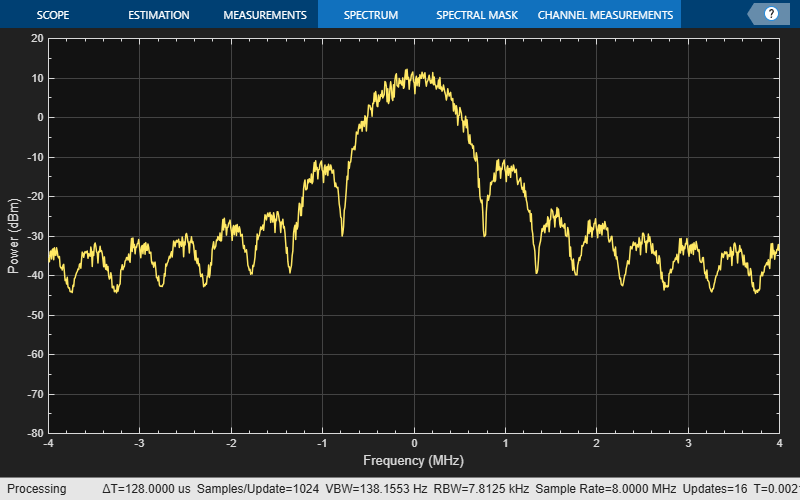# bleWaveformGenerator

Generate Bluetooth LE PHY waveform

Since R2019b

## Syntax

``waveform = bleWaveformGenerator(message)``
``waveform = bleWaveformGenerator(message,Name,Value)``

## Description

example

````waveform = bleWaveformGenerator(message)` generates `waveform`, a time-domain Bluetooth® low energy (LE) physical layer (PHY) waveform by using the input information bits, `message`.```

example

````waveform = bleWaveformGenerator(message,Name,Value)` also specifies options using one or more name-value pair arguments. For example, `'Mode','LE2M'` specifies the generating mode value of the desired Bluetooth LE waveform.```

## Examples

collapse all

Create an input message column vector of length 2056 containing random binary values. Set the symbol rate to default value.

```message = randi([0 1],2056,1); symbolRate = 1e6;```

Generate the Bluetooth LE waveform.

`waveform = bleWaveformGenerator(message);`

Create a spectrum analyzer System object to display the frequency spectrum of the generated Bluetooth LE waveform. Set the sample rate of the frequency spectrum.

```scope = spectrumAnalyzer; scope.SampleRate = 8*symbolRate;```

Plot the Bluetooth LE waveform.

`scope(waveform);`Create an input message column vector of length 640 containing random binary values.

`message = randi([0 1],640,1);`

Specify the values of the generating PHY mode and samples per symbol. Set symbol rate to the default value.

```phyMode = "LE125K"; sps = 4; symbolRate = 1e6;```

Create a spectrum analyzer System object™ to display the frequency spectrum of the generated Bluetooth LE waveform. Specify the sample rate of the frequency spectrum.

```scope = spectrumAnalyzer; scope.SampleRate = sps*symbolRate;```

Generate a Bluetooth LE waveform by specifying the modulation index and the length of the frequency pulse shape.

```waveform = bleWaveformGenerator(message,"Mode",phyMode,"SamplesPerSymbol",sps, ... "ModulationIndex",0.52,"PulseLength",3);```

Visualize the Bluetooth LE waveform.

`scope(waveform);`Specify a connection data channel protocol data unit (PDU) for angle of departure (AoD) constant tone extension (CTE).

```pduHex = '1B820127'; % Valid PDU in hexadecimal pdu = int2bit(hex2dec(pduHex),32,false);```

Generate and append cyclic redundancy check (CRC) to the PDU.

```crcGen = comm.CRCGenerator('z^24+z^10+z^9+z^6+z^4+z^3+z+1', ... 'InitialConditions',int2bit(hex2dec('555551'),24), ... 'DirectMethod',true); pduCRC = crcGen(pdu);```

Generate the Bluetooth LE waveform.

```waveform = bleWaveformGenerator(pduCRC,'ChannelIndex',36, ... 'DFPacketType','ConnectionCTE');```

## Input Arguments

collapse all

Input message bits, specified as a binary-valued column vector of numerical or logical values. This input contains the protocol data unit (PDU) and cyclic redundancy check (CRC) data. The maximum length of this value is 2088 bits.

Data Types: `double` | `logical`

### Name-Value Arguments

Specify optional pairs of arguments as `Name1=Value1,...,NameN=ValueN`, where `Name` is the argument name and `Value` is the corresponding value. Name-value arguments must appear after other arguments, but the order of the pairs does not matter.

Before R2021a, use commas to separate each name and value, and enclose `Name` in quotes.

Example: `bleWaveformGenerator(message,'Mode','LE2M','ChannelIndex',36)`

Note

For information about connection CTE and connectionless CTE direction finding packet generation, see Bluetooth Packet Structure.

PHY generating mode, specified as the comma-separated pair consisting of `'Mode'` and `'LE1M'`, `'LE2M'`, `'LE500K'`, or `'LE125K'`.

Data Types: `char` | `string`

Channel index, specified as the comma-separated pair consisting of `'ChannelIndex'`and an integer in the range [0, 39]. For data channels, this value must be in the range [0, 36]. The data-whitening block uses this value to randomize the bits.

Data Types: `double`

Samples per symbol, specified as the comma-separated pair consisting of `'SamplesPerSymbol'` and a positive integer. The function uses this value for the Gaussian frequency-shift keying (GFSK) modulation.

Data Types: `double`

Access address, specified as the comma-separated pair consisting of `'AccessAddress'` and a 32-bit column vector of numerical or logical values.

Data Types: `double` | `logical`

Type of direction finding packet, specified as the comma-separated pair consisting of `'DFPacketType'` and `'ConnectionlessCTE'`, `'ConnectionCTE'`, or `'Disabled'`.

Data Types: `char` | `string`

Data whiten status, specified as `'On'` or `'Off'`. To perform whitening on message, set this value to `'On'`.

Data Types: `char` | `string`

Modulation index, specified as a scalar in the range [0.45, 0.55]. This input specifies the modulation index that this function uses while performing GFSK modulation.

Data Types: `double`

Length of the frequency pulse shape, specified as an integer in the range [1, 4]. Units are in symbol intervals.

Data Types: `double`

## Output Arguments

collapse all

Output time-domain waveform, returned as a complex-valued column vector of size Ns-by-1, where Ns represents the number of time-domain samples. The function returns this output in the form of complex in-phase quadrature (IQ) samples. If you specify the `Mode` input as `'LE1M'` or `'LE2M'`, this output includes an additional 40 bits containing a combination of the access address and preamble length. For more information, see Bluetooth Packet Structure.

Data Types: `double`

 Bluetooth Technology Website. “Bluetooth Technology Website | The Official Website of Bluetooth Technology.” Accessed November 22, 2021. https://www.bluetooth.com/.

 Bluetooth Special Interest Group (SIG). "Bluetooth Core Specification." Version 5.3. https://www.bluetooth.com/.